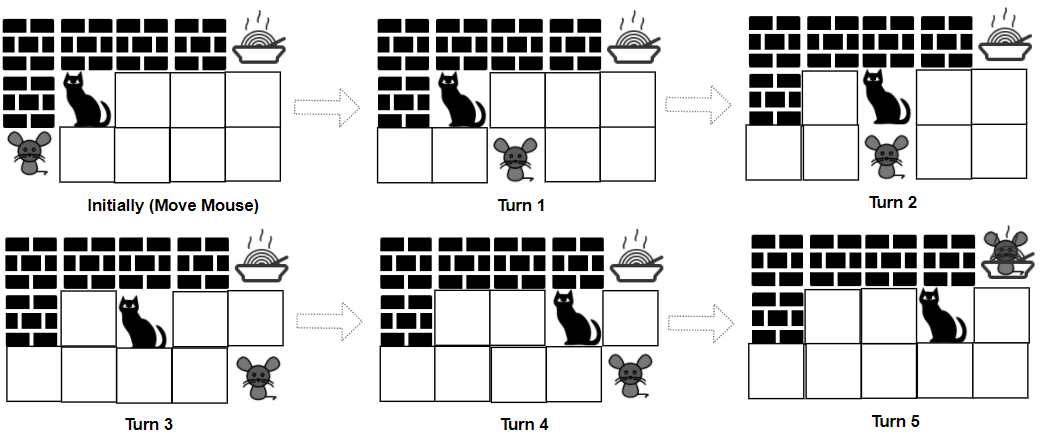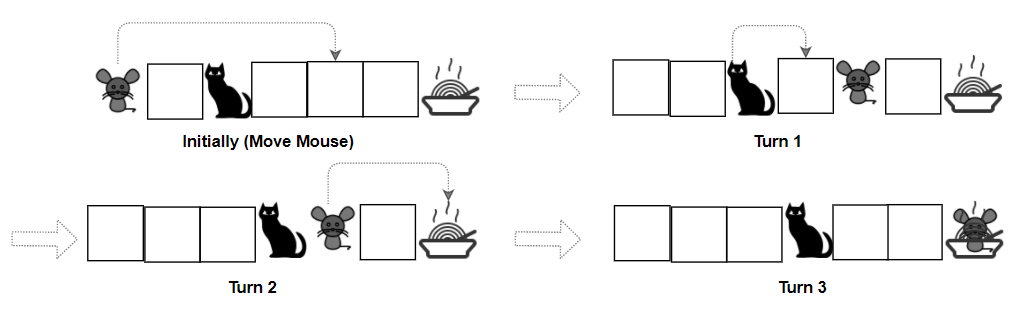Formatted question description: https://leetcode.ca/all/1728.html

# 1728. Cat and Mouse II

Hard

## Description

A game is played by a cat and a mouse named Cat and Mouse.

The environment is represented by a grid of size rows x cols, where each element is a wall, floor, player (Cat, Mouse), or food.

• Players are represented by the characters 'C'(Cat), 'M'(Mouse).
• Floors are represented by the character '.' and can be walked on.
• Walls are represented by the character '#' and cannot be walked on.
• Food is represented by the character 'F' and can be walked on.
• There is only one of each character 'C', 'M', and 'F' in grid.

Mouse and Cat play according to the following rules:

• Mouse moves first, then they take turns to move.
• During each turn, Cat and Mouse can jump in one of the four directions (left, right, up, down). They cannot jump over the wall nor outside of the grid.
• catJump, mouseJump are the maximum lengths Cat and Mouse can jump at a time, respectively. Cat and Mouse can jump less than the maximum length.
• Staying in the same position is allowed.
• Mouse can jump over Cat.

The game can end in 4 ways:

• If Cat occupies the same position as Mouse, Cat wins.
• If Cat reaches the food first, Cat wins.
• If Mouse reaches the food first, Mouse wins.
• If Mouse cannot get to the food within 1000 turns, Cat wins.

Given a rows x cols matrix grid and two integers catJump and mouseJump, return true if Mouse can win the game if both Cat and Mouse play optimally, otherwise return false.

Example 1:Input: grid = [”####F”,”#C…”,”M….”], catJump = 1, mouseJump = 2

Output: true

Explanation: Cat cannot catch Mouse on its turn nor can it get the food before Mouse.

Example 2:Input: grid = [“M.C…F”], catJump = 1, mouseJump = 4

Output: true

Example 3:

Input: grid = [“M.C…F”], catJump = 1, mouseJump = 3

Output: false

Example 4:

Input: grid = [“C…#”,”…#F”,”….#”,”M….”], catJump = 2, mouseJump = 5

Output: false

Example 5:

Input: grid = [“.M…”,”..#..”,”#..#.”,”C#.#.”,”…#F”], catJump = 3, mouseJump = 1

Output: true

Constraints:

• rows == grid.length
• cols = grid[i].length
• 1 <= rows, cols <= 8
• grid[i][j] consist only of characters 'C', 'M', 'F', '.', and '#'.
• There is only one of each character 'C', 'M', and 'F' in grid.
• 1 <= catJump, mouseJump <= 8

## Solution

Use topological sort. There are five dimensions, which are the cat’s row, the cat’s column, the mouse’s row, the mouse’s column, and the turn (0 for mouse to move, and 1 for cat to move).

First, loop over grid and obtain the positions of the cat, the mouse and the food. Next, for all the positions that are not walls, obtain the neighbors of each position for the cat and the mouse. A neighbor is a positions such that it can be reached in one move.

Use a 5D array game to store the results, where game[catRow][catColumn][mouseRow][mouseColumn][turn] represents the result of the current player when the cat is at (catRow, catColumn), the mouse is at (mouseRow, mouseColumn) and the turn is turn. The element is 1 when the current player can win, and the element is -1 when the current player is determined to lose.

Do topological sort from the states where the cat and the mouse are at the same position, and the states where either the cat or the mouse is at the position of the food.

Finally, return whether game[startCatRow][startCatColumn][startMouseRow][startMouseColumn] equals 1, where the dimensions’ meanings were mentioned above.

class Solution {
int rows, columns;

public boolean canMouseWin(String[] grid, int catJump, int mouseJump) {
rows = grid.length;
columns = grid.length();
int[][][][][] degrees = new int[rows][columns][rows][columns];
int startCatRow = -1, startCatColumn = -1, startMouseRow = -1, startMouseColumn = -1, foodRow = -1, foodColumn = -1;
for (int i = 0; i < rows; i++) {
String row = grid[i];
for (int j = 0; j < columns; j++) {
if (row.charAt(j) == 'C') {
startCatRow = i;
startCatColumn = j;
} else if (row.charAt(j) == 'M') {
startMouseRow = i;
startMouseColumn = j;
} else if (row.charAt(j) == 'F') {
foodRow = i;
foodColumn = j;
}
}
}
for (int i = 0; i < rows; i++) {
String row = grid[i];
for (int j = 0; j < columns; j++) {
if (row.charAt(j) != '#') {
for (int k = 0; k < rows; k++) {
String row2 = grid[k];
for (int m = 0; m < columns; m++) {
if (row2.charAt(m) != '#') {
degrees[i][j][k][m] = getNeighbors(grid, k, m, mouseJump).size();
degrees[i][j][k][m] = getNeighbors(grid, i, j, catJump).size();
}
}
}
}
}
}
int[][][][][] game = new int[rows][columns][rows][columns];
Queue<int[]> queue = new LinkedList<int[]>();
for (int i = 0; i < rows; i++) {
String row = grid[i];
for (int j = 0; j < columns; j++) {
if (row.charAt(j) != '#' && row.charAt(j) != 'F') {
game[i][j][i][j] = -1;
game[i][j][i][j] = 1;
queue.offer(new int[]{i, j, i, j, 0});
queue.offer(new int[]{i, j, i, j, 1});
}
}
}
for (int i = 0; i < rows; i++) {
String row = grid[i];
for (int j = 0; j < columns; j++) {
if (row.charAt(j) != '#' && row.charAt(j) != 'F') {
game[foodRow][foodColumn][i][j] = -1;
game[i][j][foodRow][foodColumn] = -1;
queue.offer(new int[]{foodRow, foodColumn, i, j, 0});
queue.offer(new int[]{i, j, foodRow, foodColumn, 1});
}
}
}
while (!queue.isEmpty()) {
int[] state = queue.poll();
int catRow = state, catColumn = state, mouseRow = state, mouseColumn = state, turn = state;
if (turn == 0) {
List<int[]> neighbors = getNeighbors(grid, catRow, catColumn, catJump);
for (int[] neighbor : neighbors) {
int row = neighbor, column = neighbor;
degrees[row][column][mouseRow][mouseColumn][turn ^ 1]--;
if (game[row][column][mouseRow][mouseColumn][turn ^ 1] == 0) {
if (game[catRow][catColumn][mouseRow][mouseColumn][turn] == -1) {
game[row][column][mouseRow][mouseColumn][turn ^ 1] = 1;
queue.offer(new int[]{row, column, mouseRow, mouseColumn, turn ^ 1});
} else if (degrees[row][column][mouseRow][mouseColumn][turn ^ 1] == 0) {
game[row][column][mouseRow][mouseColumn][turn ^ 1] = -1;
queue.offer(new int[]{row, column, mouseRow, mouseColumn, turn ^ 1});
}
}
}
} else {
List<int[]> neighbors = getNeighbors(grid, mouseRow, mouseColumn, mouseJump);
for (int[] neighbor : neighbors) {
int row = neighbor, column = neighbor;
degrees[catRow][catColumn][row][column][turn ^ 1]--;
if (game[catRow][catColumn][row][column][turn ^ 1] == 0) {
if (game[catRow][catColumn][mouseRow][mouseColumn][turn] == -1) {
game[catRow][catColumn][row][column][turn ^ 1] = 1;
queue.offer(new int[]{catRow, catColumn, row, column, turn ^ 1});
} else if (degrees[catRow][catColumn][row][column][turn ^ 1] == 0) {
game[catRow][catColumn][row][column][turn ^ 1] = -1;
queue.offer(new int[]{catRow, catColumn, row, column, turn ^ 1});
}
}
}
}
}
return game[startCatRow][startCatColumn][startMouseRow][startMouseColumn] == 1;
}

public List<int[]> getNeighbors(String[] grid, int row, int column, int maxJump) {
List<int[]> neighbors = new ArrayList<int[]>();
int[][] directions = { {-1, 0}, {1, 0}, {0, -1}, {0, 1} };
for (int[] direction : directions) {
int curRow = row, curColumn = column;
for (int i = 1; i <= maxJump; i++) {
curRow += direction;
curColumn += direction;
if (curRow < 0 || curRow >= rows || curColumn < 0 || curColumn >= columns || grid[curRow].charAt(curColumn) == '#')
break;